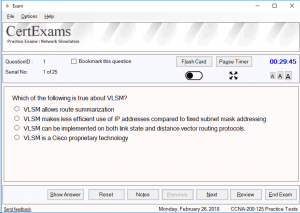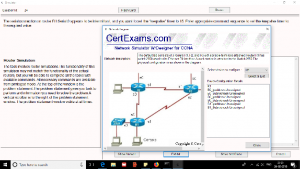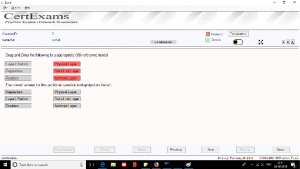# CCNA Exam Sim

Share

Certexams.com CCNA Exam Sim is among the most advanced practice tests for CCNA. Recently, practice tests have become backbone of exam preparation due to the fact that 1) They significantly improve the chances of passing the actual exam on the first attempt, 2) They provide an unavoidable means of exam preparation by simulating the actual exam environment, 3) They are available at a fraction of the cost of actual exam, 4) Useful as last minute preparation tool for self assessment.

The practice exams available from Certexams.com consists of 500+ questions with answers and flashcard explanation for each question. The question types available include MCQs, Simlets, Testlets, and Simulation type questions. The examples of each of these questions are showing in the figures below:

1.MCQ Type Question:
MCQ, short for Multiple Choice question, is the most widely used question type in objective type questions. Most of the computer based exams are made up of this type of questions. Here, typically 4 to 6 answers options are give, wherein the candidate has to select one or more best answers. Note that the answer choices are usually close enough that one needs to analyze the question and the given answers carefully before answering the question. Single answer choice questions are called Multiple Choice Single Answer (MCSA) questions, and multiple answer (more than one right answer) questions are called Multiple Choice Multi Answers (MCMA) questions. Expect both types of questions in the CCNA exam.

2. Testlet Type Question: The testlet type question provides are usually scenario based. You will be given a scenario and asked two or more questions (usually 3-5) from the given scenariio. The scenario is usually accompanied by a picture that provides a network diagram for which the scenario pertains. Though Cisco doesn’t say how much weight is given to scenario based questions, it difficult to pass the exam without having to answer such type of questions. Hence, it is required that you prepare for such questions thoroughly before going to the exam. The CCNA practice exam covers several such questions for thorough preparation.

3. Simlet Type Question:The Simlet type questions are similar to Testlet questions in the sense that there is a scenario which the candidate needs to understand. However, Simlet questions provide a network simulation in which a network diagram is provided along with active command interface. Typically, you would be able to type and understand the network configuration by querying each of the network device. By verifying the network configuration as given by the devices show run command (for example), you would be able to answer the questions in the simlet. You will be tested for identifying and interpreting various parameter values in the IOS command output. Usually, you don’t need to configure any devices. Just reading the configuration using appropriate command should suffice to answer the given questions.

4. Router Simulation Type Questions: It is the most difficult of all types of questions.  Here, you will be asked to troubleshoot and correct any configuration errors. All necessary commands to correct the configuration on the virtual network are provided. You need to type in the necessary command(s) on one or more routers to fix the configuration errors.

5. Drag-n-Drop Type Question:This question type, as the name implies, consists of two sets of parameters that you need to match. For example, one set may represent the wireless standards and the other set may represent the speeds. You may be asked to match the standard with corresponding speeds.

Among all these questions types, Simulation type questions are considered to be a bit difficult to answer as it requires not only understanding the network scenario, but also the familiarity with using commands as appropriate to troubleshoot the problem. In this context, CCNA Net Sim would be very useful